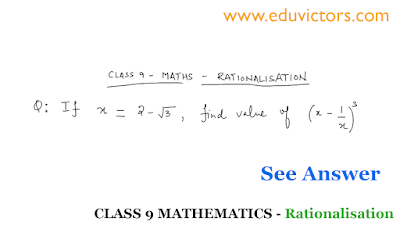## Pages

Showing posts with label class9-maths. Show all posts
Showing posts with label class9-maths. Show all posts

# National Mathematics Day

National Mathematics Day is observed annually on December 22nd to mark the anniversary of the eminent mathematician Srinivasa Ramanujan's birth.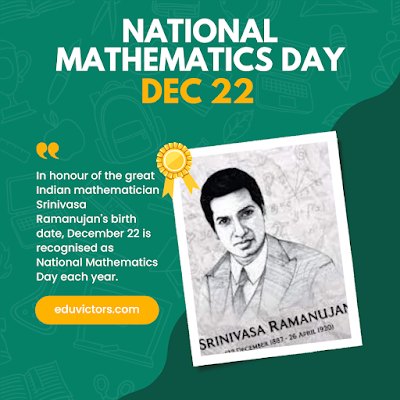# Class 9 Maths Properties of Quadrilateral Figures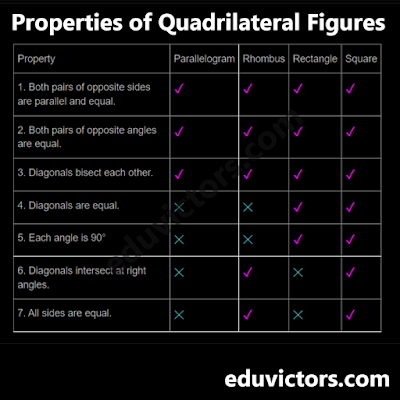# CBSE Class 9 Maths - Half Yearly Examination Question Paper

Even though you are familiar with the concepts in mathematics, you still need to practise in order to cement the notions in your memory. Some students find math to be a very intriguing subject, while others detest it because it is only based on numbers. Practice is one of the best strategies to overcome this phobia of math. The best strategy to reinforce the concepts is to practise on CBSE Sample Papers for Class 9 Math. It helps students to have a real-time experience of question paper pattern, so that they can prepare accordingly.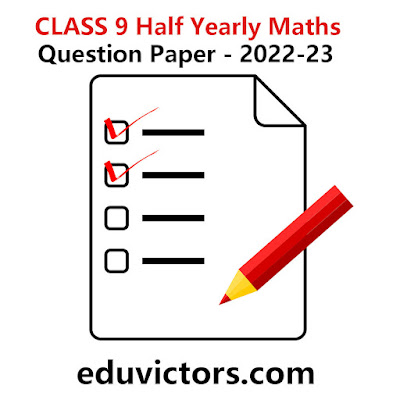# Maths Tip - 1 : Find a rational number and an irrational number between two rational numbers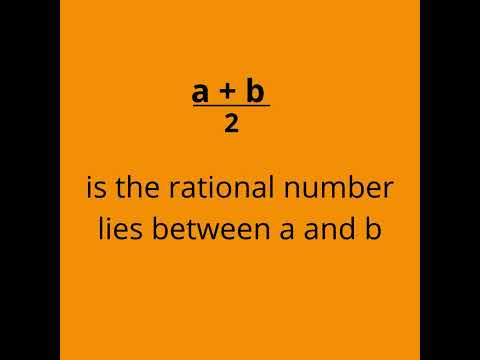# CLASS IX-X: DELETED CHAPTERS IN MATHS AND SCIENCE IN SYLLABUS (2022-23)

Please note the Board will conduct the annual scheme of assessment at the end of the Academic Session 2022-23

Class 9 Science

Deleted Chapters:

- DIVERSITY IN LIVING ORGANISMS

- NATURAL RESOURCES

- WHY DO WE FALL ILL?

Chapter: NATURAL RESOURCES will be taught in the class and  students are encouraged to prepare a brief write up on any concept of this chapter in their Portfolio (internal assessment)For Class 7 / Class 8 / Class 9

The worksheet will help you understand the basic definition of Rational and its properties.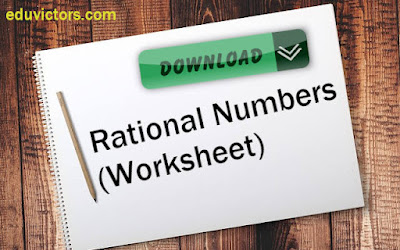# CBSE Class 9 - Circles - NCERT Exercise 10.2 + Questions

Question 1: Recall that two circles are congruent if they have the same radii. Prove that equal chords of congruent circles subtend equal angles at their centres.

Given: Circle C (A, r) and C (P, r) are two congruent circles such that BC = QR.

To prove: ∠BAC = ∠QPR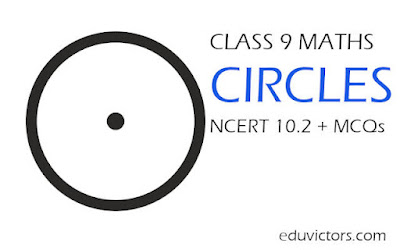# CBSE Class 9 - Maths - Polynomials MCQs

Choose the correct option from the choices given in each question.

Q1: Which one of the following is incorrect about polynomials?

(a) A monomial contains one non-zero term.

(b) A polynomial is an algebraic expression.

(c) Exponents of a polynomial can be irrational.

(d) Coefficients of a polynomial can be irrational.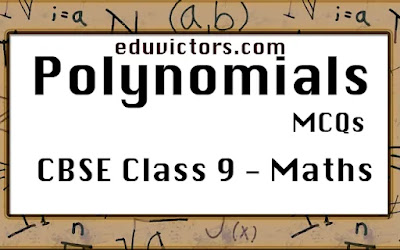# National Mathematics Day

India celebrates National Mathematics Day on December 22 every year. The day marks the birth anniversary of famous mathematician Srinivasa Ramanujan.

*Typo in the graphic new = knew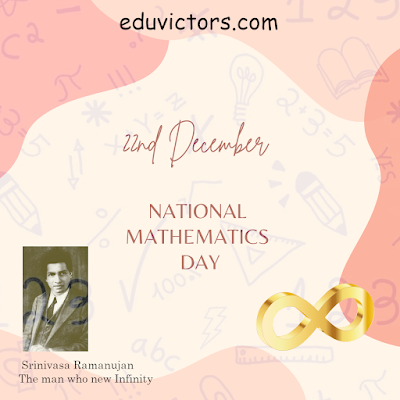# CBSE Class 9 Maths - Linear Equations in Two Variables (MCQs)

Q1: A linear equation of two variables x and y can be represented as ax + by = c, where a, b, and c are:

(A) Rational numbers

(B) Irrational numbers

(C) Integers

(D) Real Numbers

Q2: The graph of the equation of the form y = kx is a line which?

(A) May pass through the origin.

(B) Always passes through the origin.

(C) Will not pass through origin

(D) Will be parallel to x-axis## Tuesday, 13 July 2021

### Class 9 Maths - Important Identities (#class9Maths)(#eduvictors)

Class 9 Maths - Important Identities

(a+b)² = a² + 2ab+ b²

(a–b)² = a² –2ab + b²

a² – b² = (a+b)(a–b)# CBSE Class 9  Maths - Number Systems - Very Short Answer Based Questions

Q1: Simplify $\sqrt{32}$ ?

$\sqrt{32} = \left ( 32 \right )^{\frac{1}{4}}$

$= \left ( 2^5 \right )^{\frac{1}{4}}$

$= \left ( 2^4 . 2^1 \right )^{\frac{1}{4}}$

$= \left ( 2^4 \right )^{\frac{1}{4}}.(2)^{\frac{1}{4}}$

$= 2.\sqrt{2}$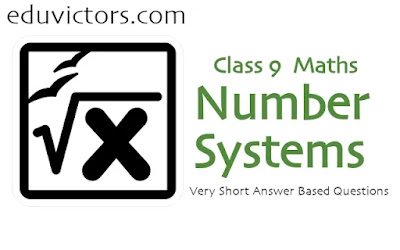# CBSE Class 9 - Maths - Real Numbers (MCQs)

Choose the correct option in each of the following questions:

Q1. Which of the following is a rational number?

(a) 2√3

(b) π

(c) 0

(d) √3 + 1

Q2. Every point on a number line represents

(a) a rational number

(b) a natural number

(c) an irrational number

(d) a real number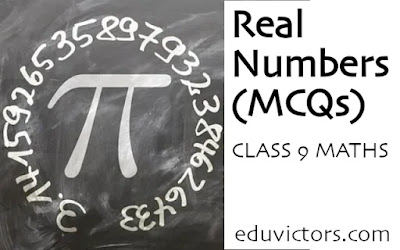# Class 9 Maths Annual Sample Question Paper  (Set-1) 2020-21

Learning is a continual process. To improve scores, the best way is to learn and practice. Solving question papers help students to evaluate their knowledge and familarise with different types of question patterns being asked in examination.

Eduvictors provides CBSE Previous Year Papers and Sample Question Papers for Class 9 Maths, Science, Social, English, Hindi and Sanskrit with/without solutions to help students in their board exam preparation.

Here attached the Maths Sample Question Paper (2020-2021).# Class 9 - Maths - Quadrilaterals (Worksheet-4)

Q1: ABCD is a parallelogram such that its diagonals are equal. What is the measure of ∠ABC?

Answer: Since diagonals are equal, it must be a rectangle or a square. ∴ ∠ABC = 90°

Q2: Is rhombus a kite or a parallelogram?

Q3: In a parallelogram ABCD, if ∠C = 80° then what is the measure of ∠A?

Answer: In a parallelogram, opposite angles are equal, ∴ ∠A = ∠C = 80°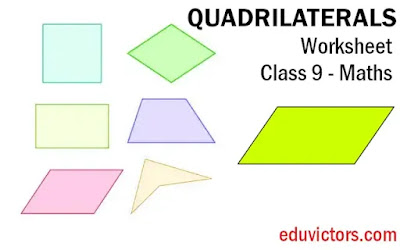# CBSE Class 9 Maths - Circles - Quiz

MCQs (Q1 - Q10)

Q1. The diameter of a circle is also a

(a) secant

(b) tangent

(d) chord

Q2. An equilateral triangle ABC is inscribed in a circle with centre O.The measure of ∠BOC is

(a) 110⁰

(b) 100⁰

(c) 120⁰

(d) 130⁰# Lines and Angles (Important Terms and Definitions)

CBSE Class 9 - Maths

1. Two angles are called adjacent angles, if

(i) they have the same vertex,

(ii) they have a common arm and

(iii) their non-common arms are on either side of the common arm.

2. Two adjacent angles are said to form a linear pair of angles, if their non-common arms are two opposite rays.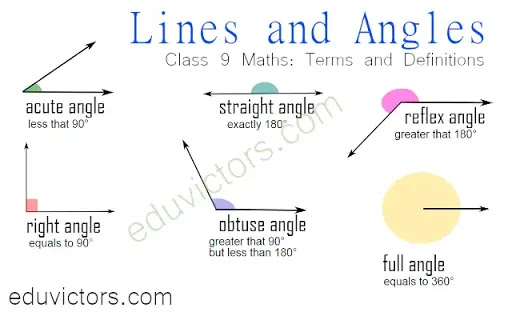# Triangles - Concept Points

Class 9 - Mathematics

1. A plane figure bounded by three line segments is called a triangle.

2. A triangle can be:
- equilateral (all three sides are equal)
- isosceles (any two sides are equal)
- scalene (all three sides are unequal)

3. A triangle in which one of the angles measures  90° is called a right-angled triangle or simply a right triangle.

4. The sum of the angles of a triangle is always 180°

5. Two figures are congruent, if they are of same shape and same size. Congruent triangles are exactly identical.## CBSE Class 9 - Maths - Real Numbers - Problems on Rationalisation (Part-5)

The part-5 video gives a solution to a typical problem on rationalisation. This type of problem is commonly asked in question papers. The first step to solve this kind of problem is to rationalise each term individually. The video covers the solution for a long type of answer based question. In this series, we'll try to present solutions for more HOTS and typical problems for students of mathematics of class 9 For study Notes, Worksheets, Question Papers and Assignments for CBSE Class 6 - 12, do check our website www.eduvictors.com## CBSE Class 9 - Maths - Real Numbers - Problems on Rationalisation (Part-4)

The objective of rationalizing a denominator is to make it easier to understand what the quantity really is by removing irrationals from the denominators. The video series provides solutions for typical problems related to rationalization of irrational numbers covered under chapter real numbers. The video covers the solution for a long type of answer based question. In this series, we'll try to present solutions for more HOTS and typical problems for students of mathematics of class 9 For study Notes, Worksheets, Question Papers and Assignments for CBSE Class 6 - 12, do check our website www.eduvictors.com